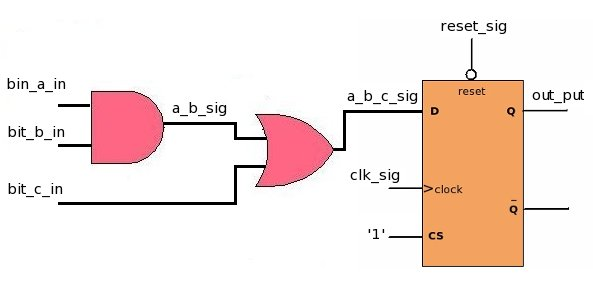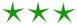black nike shoes roshe cheapnicesports.com

# Coding Sequence

In general purpose languages like C/C++, the code is executed line after line. But in vhdl component's connection is important then the code placement. You can place components any where, but their connection between them will decide its final behavior.

Lets take an example and try to understand it ...The above circuit is coded in three different ways and in each of them components are placed differently.```a_b_sig<= bit_a_in AND bit_b_in; a_b_c_sig<= a_b_sig OR bit_c_in; process: (clk_sig, reset_sig) begin if(reset_sig = '0')then out_put <= '0'; elsif(clk_sig 'event AND clk_sig = '1') then out_put <= a_b_c_sig; end if; end process ; ``````a_b_sig<= bit_a_in AND bit_b_in; process: (clk_sig, reset_sig) begin if(reset_sig = '0')then out_put <= '0'; elsif(clk_sig 'event AND clk_sig = '1') then out_put <= a_b_c_sig; end if; end process ; a_b_c_sig<= a_b_sig OR bit_c_in; `````` process: (clk_sig, reset_sig) begin if(reset_sig = '0')then out_put <= '0'; elsif(clk_sig 'event AND clk_sig = '1') then out_put <= a_b_c_sig; end if; end process ; a_b_c_sig<= a_b_sig OR bit_c_in; a_b_sig<= bit_a_in AND bit_b_in; ```

All three codes are same and will represents same hardware. Thus in VHDL how components are placed in file is not important, only important thing is how these components are connected.